Correlation

Sum of Squares

We introduced a notation earlier in the course called the sum of squares. This notation was the SS notation, and will make these formulas much easier to work with.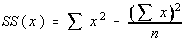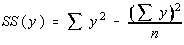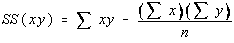Notice these are all the same pattern,

SS(x) could be written as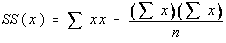Also note that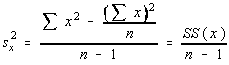Pearson's Correlation Coefficient

There is a measure of linear correlation. The population parameter is denoted by the greek letter rho and the sample statistic is denoted by the roman letter r.

Here are some properties of r

• r only measures the strength of a linear relationship. There are other kinds of relationships besides linear.
• r is always between -1 and 1 inclusive. -1 means perfect negative linear correlation and +1 means perfect positive linear correlation
• r has the same sign as the slope of the regression (best fit) line
• r does not change if the independent (x) and dependent (y) variables are interchanged
• r does not change if the scale on either variable is changed. You may multiply, divide, add, or subtract a value to/from all the x-values or y-values without changing the value of r.
• r has a Student's t distribution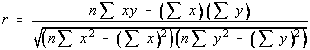Here is the formula for r. Don't worry about it, we won't be finding it this way. This formula can be simplified through some simple algebra and then some substitutions using the SS notation discussed earlier.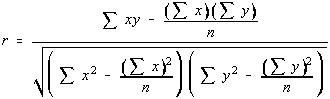If you divide the numerator and denominator by n, then you get something which is starting to hopefully look familiar. Each of these values have been seen before in the Sum of Squares notation section. So, the linear correlation coefficient can be written in terms of sum of squares.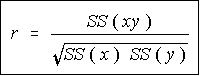This is the formula that we would be using for calculating the linear correlation coefficient if we were doing it by hand. Luckily for us, the TI-82 has this calculation built into it, and we won't have to do it by hand at all.

Hypothesis Testing

The claim we will be testing is "There is significant linear correlation"

The Greek letter for r is rho, so the parameter used for linear correlation is rho

• H0: rho = 0
• H1: rho <> 0

r has a t distribution with n-2 degrees of freedom, and the test statistic is given by: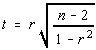Now, there are n-2 degrees of freedom this time. This is a difference from before. As an over-simplification, you subtract one degree of freedom for each variable, and since there are 2 variables, the degrees of freedom are n-2.

This doesn't look like our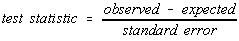If you consider the standard error for r is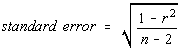the formula for the test statistic is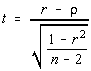, which does look like the pattern we're looking for.

Remember that

Hypothesis testing is always done under the assumption that the null hypothesis is true.

Since H0 is rho = 0, this formula is equivalent to the one given in the book.
Additional Note: 1-r2 is later identified as the coefficient of non-determination

Hypothesis Testing Revisited

If you are testing to see if there is significant linear correlation (a two tailed test), then there is another way to perform the hypothesis testing. There is a table of critical values for the Pearson's Product Moment Coefficient (PPMC) given in the text book. The degrees of freedom are n-2.

Depending on the textbook you're using, you may be required to look up either n or the df in the table. Be sure to look at the table before you use it or you may get wrong critical values. In the Triola text, it is the sample size, n, that you look up. In the Bluman text, it is the df=n-2 that you look up.

The test statistic in this case is simply the value of r. You compare the absolute value of r (don't worry if it's negative or positive) to the critical value in the table. If the test statistic is greater than the critical value, then there is significant linear correlation. Furthermore, you are able to say there is significant positive linear correlation if the original value of r is positive, and significant negative linear correlation if the original value of r was negative.

There are three valid conclusions

• There is no significant linear correlation
• There is significant positive linear correlation
• There is significant negative linear correlation

Use the first one if you fail to reject the null hypothesis, that is, your test statistic is not bigger than the critical value.

Use the second one if you reject the null hypothesis (your test statistic is bigger than the critical value) and your test statistic is positive.

Use the last one if you reject the null hypothesis (your test statistic is bigger than the critical value) and your test statistic is negative.

Using the table to look up the critical values is the most common technique. However, the first technique, with the t-value must be used if it is not a two-tail test, or if a different level of significance (other than 0.01 or 0.05) is desired.

Puzzle Time?

Okay, here's a puzzle for you. The first question is ...

If two things are not equal, does that mean one's bigger?

And the second question is similar ...

If one thing is bigger than another, does that mean they're not equal?

Now, the real kicker. One of the answers is "yes", and the other is "no".

Grab your aspirin and think about the statements in the context of hypothesis testing.

Saying that two things are not equal means that you have rejected the null hypothesis in a two-tail test. So, if the level of significance is 0.10, then with a two-tail test, you have 0.05 on the right side. So, if you say "two things aren't equal" because you reject the null hypothesis, then your probability-value must have been less than 0.05.

To say that one thing is bigger means to reject the null hypothesis with a right-tail test. So, if the level of significance is 0.10, then with a right tail test, you have all 0.10 of that on the right side. So, if you say "one thing is bigger" because you reject the null hypothesis, then your probability value must be less than 0.10.

Still with me? Let's analyze the statements above.

If two things are not equal, does that mean one's bigger?
Our condition is that two things are not equal. That means that the p-value is less than 0.05. Can we say that one is bigger? To do so would require that the p-value be less than 0.10. So, the whole question comes down to the following: If the p-value is less than 0.05, is it less than 0.10. Yes. So, we can say that if two things aren't equal, one is definitely bigger.
If one thing is bigger than another, does that mean they're not equal?
Our condition is that one thing is bigger than another. That means that the p-value is less than 0.10. Can we say they're not equal? To do so would require that the p-value be less than 0.05. So, the whole question comes down to the following: If the p-value is less than 0.10, is it less than 0.05? Not necessarily! It could be 0.07. So, no, we cannot say that just because one thing is bigger, it means they're not equal.

What the @*&#^@* does that have to do with anything?

When we use the table to look up the critical values, it only gives us the critical values for a two-tail test. That is, we're testing that either there is significant linear correlation or that there isn't (two-tail). We're not testing for positive linear correlation (right-tail) or negative linear correlation (left-tail).

How then can we get by with our conclusion that uses the word positive or the word negative in it? If we reject the claim that two things are equal (rho = 0), then one has to be bigger. So, either rho is greater than zero (positive correlation) or zero is bigger than rho (negative correlation).

Causation

If there is a significant linear correlation between two variables, then one of five situations can be true.

• There is a direct cause and effect relationship
• There is a reverse cause and effect relationship
• The relationship may be caused by a third variable
• The relationship may be caused by complex interactions of several variables
• The relationship may be coincidental

Common Errors

There are some common errors that are made when looking at correlation.

• Avoid concluding causation. We just got through talking about causation. Just because there is a linear relationship doesn't mean that one thing caused the other. It could be any of the five situations above.
• Avoid data based on rates or averages. Variation is suppressed when using a rate or an average. Remember the central limit theorem? The variance of the sample means was the variance of the population divided by the sample size. So, if you work with averages, the variances are smaller and you might be able to find linear relationships that are significant when they would not be if the original data was used.
• Watch out for linearity. All that we're testing here is the strength of a linear relationship. There are other kinds of relationships. In algebra, we talk about linear, quadratic, cubic, quartic, exponential, logarithmic, Gaussian (bell shaped), logistics, and power models. A scatter plot is a good way to look for patterns.